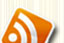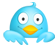October 25, 2011 PHP

### Seconds to String

This function will return the duration of the given time period in days, hours, minutes and seconds.

e.g. secondsToStr(1234567) would return “14 days, 6 hours, 56 minutes, 7 seconds”
[code type=php]
<?php
function secondsToStr(\$secs) {
if(\$secs>=86400){\$days=floor(\$secs/86400);\$secs=\$secs%86400;\$r=\$days.’ day’;if(\$days<>1){\$r.=’s’;}if(\$secs>0){\$r.=’, ‘;}}
if(\$secs>=3600){\$hours=floor(\$secs/3600);\$secs=\$secs%3600;\$r.=\$hours.’ hour’;if(\$hours<>1){\$r.=’s’;}if(\$secs>0){\$r.=’, ‘;}}
if(\$secs>=60){\$minutes=floor(\$secs/60);\$secs=\$secs%60;\$r.=\$minutes.’ minute’;if(\$minutes<>1){\$r.=’s’;}if(\$secs>0){\$r.=’, ‘;}}
\$r.=\$secs.’ second’;if(\$secs<>1){\$r.=’s’;}
return \$r;
}

?>
[/code]

Tags: